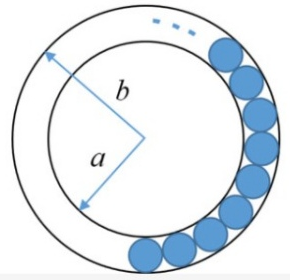# GATE | GATE CS 2020 | Question 8

The figure below shows an annular ring with outer and inner as b and a, respectively. The annular space has been painted in the form of blue colour circles touching the outer and inner periphery of annular space. If maximum n number of circles can be painted, then the unpainted area available in annular space is _________ .(A) π[(b2−a2)−(n/4)(b−a)2]
(B) π[(b2−a2)−n(b−a)2]
(C) π[(b2−a2)+(n/4)(b−a)2]
(D) π[(b2−a2)+n(b−a)2]

Explanation: Area of 1 blue circle,

`= π((b-a)/2)2`

Hence, area of n blue circles,

`= nπ((b-a)/2)2`

Now, Area of annular ring

`= πb2 – πa2 `

```= (Area of annular ring) – (Area of n blue color circles)
= ( πb2 – πa2 ) - ( nπ((b-a)/2)2 )
= π( b2 – a2 ) - π( (n/4)(b-a)2 )
= π[(b2−a2)−(n/4)(b−a)2] ```

Option (A) is correct.

Quiz of this Question

My Personal Notes arrow_drop_up
Article Tags :

1

Please write to us at contribute@geeksforgeeks.org to report any issue with the above content.